# Differential Amplifier or Voltage Subtractor Circuit

Published  December 11, 2018   0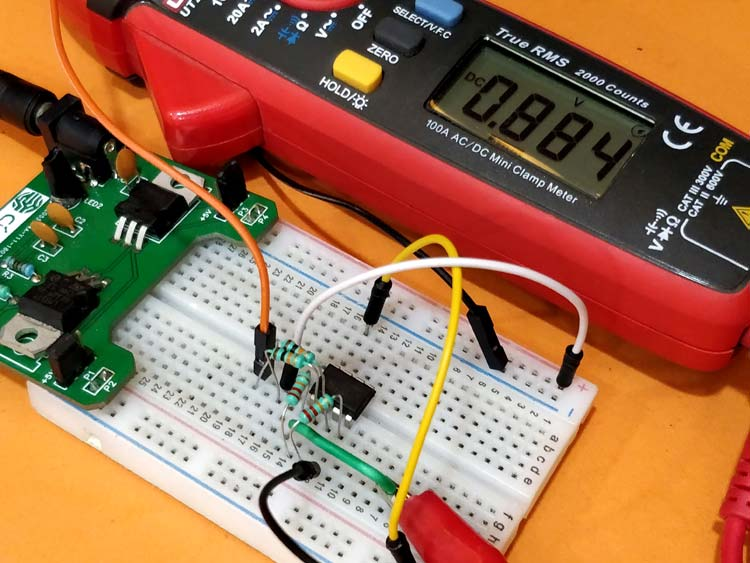Op-Amps were originally developed for Analog mathematical calculations, since then today they have proved to be useful in many design applications. As my professor ones rightly said, op-amps are arithmetic voltage calculators, they can perform addition of two given voltage values using Summing Amplifier circuit and difference between two voltage values using a Differential Amplifier. Apart from this the Op-Amp is also commonly used as Inverting Amplifiers and Non-Inverting Amplifiers.

We have already learnt how we can use an Op-Amp as a Voltage Adder or Summing Amplifier, so in this tutorial we will learn how to use op-amp as a Differential amplifier to find the voltage difference between two voltage values. It is also called the Voltage Subtractor. We will also try the voltage subtractor circuit on a breadboard and check if the circuit is working as expected.

### Basics of Op-Amp

Before we dive into the differential Op-amps, let’s quickly run through the basics of Op-Amp. An Op-Amp is a five terminal device (single package) with two terminals (Vs+, Vs-) for powering the device. Of the remaining three terminals two (V+, V-) are used for signals which are called the Inverting and Non-Inverting terminal and the remaining one (Vout) is the output terminal. The basic symbol of an Op-Amp is shown below.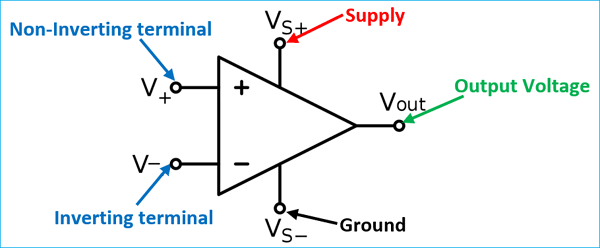The working of an Op-Amp is very simple, it takes in the different voltage from two pins (V+, V-), amplifies it by a Gain value and gives it as the output voltage (Vout). The gain of an Op-Amp can be very high making it suitable for audio applications. Always remember that the input voltage of the Op-Amp should be less than its operating voltage. To learn more about op-amp, check its application in various op-amp based circuits.

For an Ideal Op-Amp the input impedance will be very high, that is no current will flow in or out the Op-Amp through the Input pins (V+, V-). To understand the working of op-amp we can broadly categorize the op-amp circuits as open loop and closed loop.

### Op-amp Open loop circuit (Comparators)

In an open loop op-amp circuit, output pin (Vout) is not connected with any of the input pins, that is no feedback is provided. In such open-loop conditions the op-amp works as a comparator. A simple op-amp comparator is shown below. Notice that the Vout pin is not connected with input pins V1 or V2.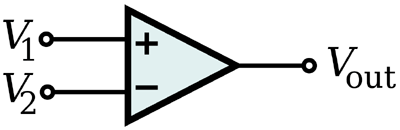In this condition, if the voltage supplied to V1 is greater than V2 the output Vout will go high. Likewise if the voltages supplied to V2 are greater than V1 then output Vout will go low.

### Op-amp closed loop circuit (Amplifiers)

In a closed loop op-amp circuit the output pin of the op-amp is connected with the either of the input pin to provide a feedback. This feedback is called as the closed loop connection. During closed loop an Op-amp works as an amplifier, it is during this mode an op-amp finds many useful applications like buffer, voltage follower, Inverting Amplifier, Non-Inverting amplifier, Summing amplifier, Differential amplifier, Voltage subtractor etc. If the Vout pin is connected to the Inverting terminal then it is called as negative feedback circuit (shown below) and if it connected to the Non-Inverting terminal it is called as the Positive feedback circuit.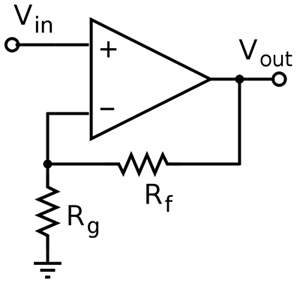### Differential Amplifier or Voltage Subtractor

Now let us get into our topic, Differential Amplifier. A differential amplifier basically takes in two voltage values, finds the difference between these two values and amplifies it. The resulting voltage can be obtained from the output pin. A basic Differential amplifier circuit is shown below.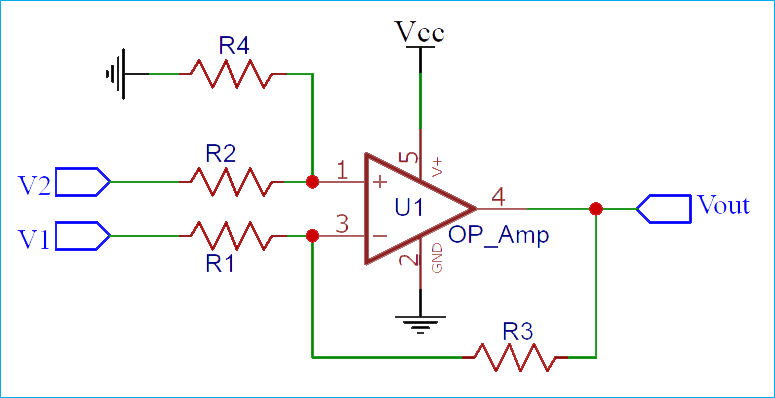But wait!, isn’t this what an Op-Amp does by default even when it has no feedback, it takes two inputs and provides their differences on the output pin. Then why do we need all these fancy resistors for?

Well yes, but op-amp when used in open loop (without feedback) will have a very high uncontrolled gain which is practically not useful. So we use the above design to set the value of gain using resistors in a negative feedback loop. In our circuit above the resistor R3 acts as a negative feedback resistor and the resistors R2 and R4 forms a potential divider. The value of gain can be set by using the right value of resistors.

### How to set the gain of a differential amplifier?

The output voltage of the differential amplifier shown above can be given by the below formula

`Vout = -V1 (R3/R1) + V2 (R4/(R2+R4))((R1+R3)/R1)`

The above formula was obtained from the transfer function of the above circuit using superposition theorem. But let’s not get much into that. We can further simplify the above equation by considering R1=R2 and R3=R4. So we will get

`Vout = (R3/R1)(V2-V1)     when R1=R2 and R3=R4`

From the above formula we can conclude that the ratio between R3 and R1 will be equal to the gain of the amplifier.

`Gain = R3/R1`

Now, let’s substitute resistors values for the above circuit and check if the circuit is working as expected.

### Simulation of Differential Amplifier circuit

The resistor value that I have chosen is 10k for R1 and R2 and 22k for R3 and R4. The circuit simulation for the same is shown below.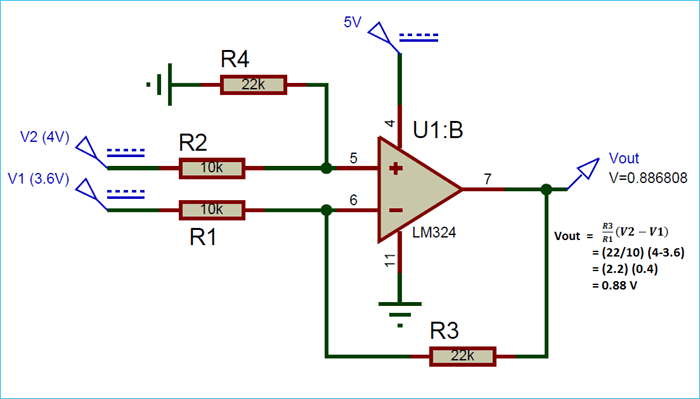For the purpose of simulation, I have supplied 4V for V2 and 3.6V for V1. The resistor 22k and 10k according the formulae will set a gain of 2.2 (22/10). So the subtraction will be be 0.4V (4-3.6) and it will be multiplied with the gain value 2.2 so the resulting voltage will be 0.88V as shown in the above simulation. Let’s also verify the same using the formula that we discussed earlier.

```Vout = (R3/R1)(V2-V1) when R1=R2 and R3=R4

= (22/10)(4-3.6)

= (2.2) x (0.4)

= 0.88v```

### Testing the Differential Amplifier Circuit on Hardware

Now to the fun part, let’s actually build the same circuit on the breadboard and check if we get are able to achieve the same results. I am using the LM324 Op-Amp to build the circuit and using the Breadboard power supply module that we built earlier. This module can provide 5V and 3.3V output, so I am using the 5V power rail to power my op-amp and the 3.3V power rail as V1. Then I used my RPS (Regulated Power Supply) to provide 3.7V to the pin V2. The difference between the voltages is 0.4 and we have a gain of 2.2 which should give us 0.88V and that is exactly what I got. The picture below shows the set-up and the multimeter with the reading 0.88V on it.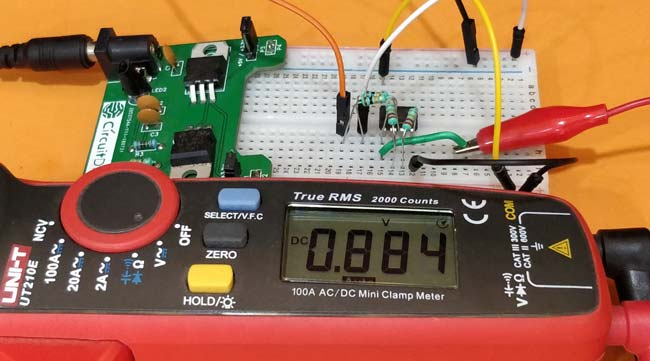This proves that our understanding of differential op-amp is correct and now we know how to design one on our own with the required gain value. The complete working can also be found in the video given below. These circuits are more often used in volume control applications.

But, since the circuit has resistors on the input voltage side (V1 and V2) it does not provide very high input impedance and also has a high common mode gain which leads to low CMRR ratio. To overcome these disadvantages there exist an improvised version of differential amplifier called the instrumentation amplifier, but let’s leave that for another tutorial.

Hope you understood the tutorial and enjoyed learning about differential amplifiers. If you have any queries leave them in the comment section or use the forums for more technical questions and faster response.

Video